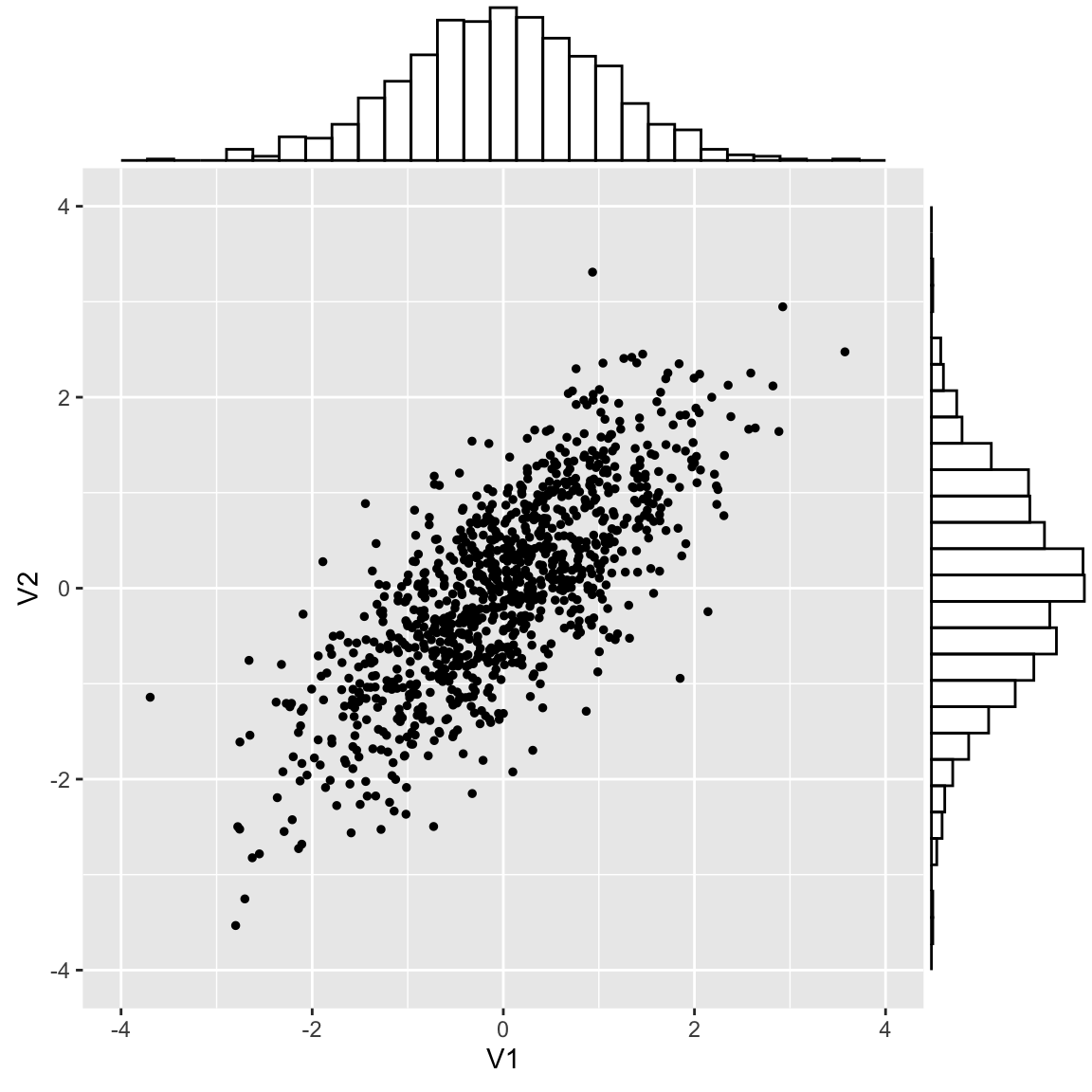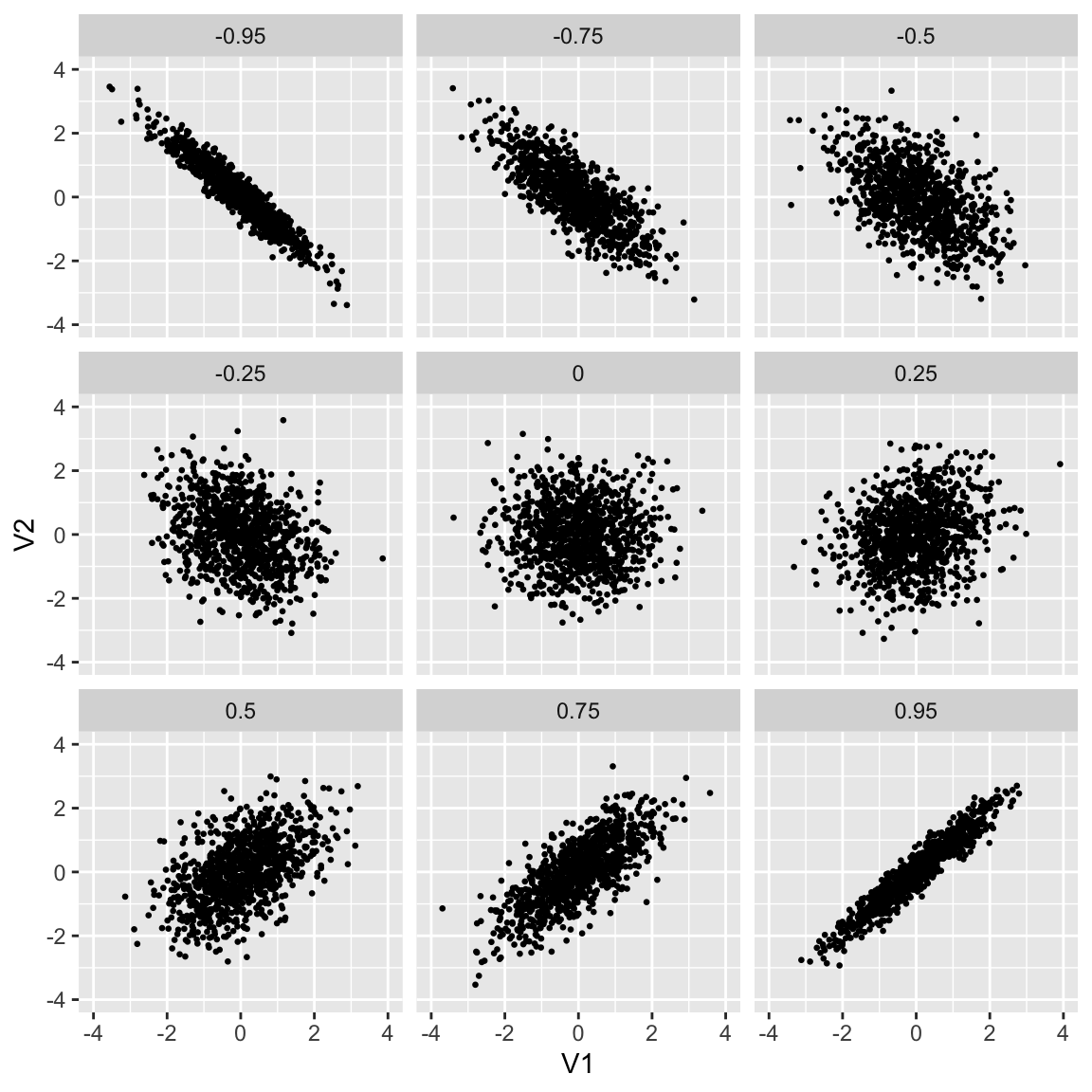# Systems Biology

## Genes work in teams

We would like to know how genes/proteins/molecules interact

These interactions can be described with a network

So we would like to find the network that represent the interactions

At first, we want to know who interacts with who

## Metabolic networks are easy/hard

Metabolic networks are directed bipartite graphs, with metabolites and reactions

If we know the stoichiometry of all reactions, we automatically know the metabolites

If we know the enzymes that can be encoded, then we know the reaction

This is the approach taken by KEGG and BioCyc

## Transcriptional regulation networks

Several parts: some genes encode transcription factors, which can bind to DNA

We simplify to a directed bipartite network: genes and binding sites

We can predict if a gene encodes a transcription factor

We can predict if a site is a binding site

But we need experiments to see which transcription factor binds to which sites

## Interaction networks

Metabolic and transcriptional networks are very important, and we need several classes to study them

Today we begin with a simpler problem: gene interaction networks

These will be undirected non-bipartite networks

They may be later extended to a directed network with more attributes

## What is interaction?

First idea: Correlation

It is a square matrix, so we can see it as an adjacency matrix

Thus, we can draw a network

## What is the network?Depending on how we define “significant correlation”, we have more or less edges

## Not too small, not too bigIf nothing is connected to nothing, we do not learn anything
The same happens if everything is connected to everything

## Three strategies

• Thresholding

• Pruning

• Regularization

## Defining a threshold

Remember that we observe the sample correlation, which is usually different from the real correlation

Sample correlation may be non-zero even if the real correlation is zero

We can use a statistical test to decide which correlations are significant

This test defines a threshold, which depends on the number of nodes

## PruningEven after thresholding, we often have spurious links

If A is correlated with B, and B is correlated with C, then there will be a small (but significant) correlation between A and C

One pruning strategy is to drop the “weakest link” of every triangle

## Pruning cyclesThis results in a network with the shape of a tree

More specifically, we get a Spanning Tree

## Assuming expression follows is NormalExpression has a random component

Genes may not be independent

## Plotting both values shows a patternThis is called a multinormal distribution

## Correlation defines the shape## Formula for multinormal

For one variable, the Normal distribution is $\frac{1}{\sqrt{2πσ^2}}\exp(-(x-μ)^2/2σ^2)$ where $$\sigma^2$$ is the variance. The multinormal distribution is $\frac{1}{\sqrt{(2π)^n\det(Σ)}}\exp(-(𝐱-𝐮)^T Σ^{-1} (𝐱-𝐮)/2)$ where $$𝐱$$ is a vector and $$Σ$$ is the covariance matrix

## The important part

Ignoring the constants, replacing $$𝐲 = 𝐱-𝐮,$$ and taking logarithms, we have that the probability depends on $𝐲^T Σ^{-1} 𝐲$ so the relationship between the genes is given by $K=Σ^{-1}$

This $$K$$ is called the precision matrix

(remember that the vector $$𝐲$$ components are the gene expressions)

## Example

\begin{aligned} K&=\begin{pmatrix} 1.00 & -0.25 & 0.0\\ -0.25 & 1.00 & 0.3\\ 0.00 & 0.30 & 1.0\\ \end{pmatrix}\\ \Sigma&=\begin{pmatrix} 1.0737 & 0.2949 & -0.0884\\ 0.2949 & 1.1799 & -0.3539\\ -0.0884 & -0.3539 & 1.1061\\ \end{pmatrix}\end{aligned}

Covariance does not reflect the real relationships

## Estimating the precision matrix

As usual, the problem is that we do not know the real covariance, only the sample covariance

Moreover, if we have less samples than genes, then the sample covariance matrix cannot be inverted

Instead, we use methods to calculate pseudo-inverses

We will see one of these methods

## Sparse matrices

One condition we can require from our solution is that each gene interacts only with a small number of genes

In general we do not expect that each of the thousand of genes interacts with most of the other thousands of genes

In other words, we expect that the adjacency matrix has mostly zeros

Matrix where the majority of the values are zero are called sparse

## Lasso in linear models

Lasso is a method used in linear models to force sparse coefficients

Instead of minimizing $$\sum_i \left(y_i - \sum_j β_j x_{ij}\right)^2,$$ the lasso method minimizes $\sum_i \left(y_i - \sum_j β_j x_{ij}\right)^2 - λ \sum_j\vert \beta_j\vert$

The parameter $$λ$$ has to be chosen by us.
Larger $$λ$$ means sparser $$β_j,$$ but bigger error

## Graphical lasso

Using the same philosophy, one way to find a sparse pseudo-inverse of $$\Sigma$$ is to find $$K$$ that minimizes $\log\det(K)-\text{tr}(Σ K) -λ\Vert K\Vert_1$

Of course, this is done by the computer for us

We only must pay attention to $$λ$$

## Graphical lasso gives a family of possible networks

Depending on the value of $$\lambda,$$ the result graphical lasso will be more or less sparse

That means that the networks will have more or less edges